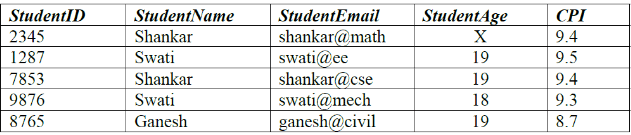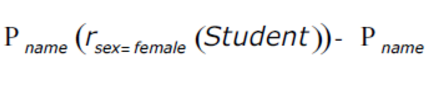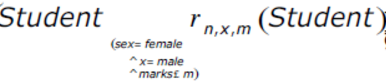Courses

# Test: Normal Forms- 2

## 20 Questions MCQ Test RRB JE for Computer Science Engineering | Test: Normal Forms- 2

Description
This mock test of Test: Normal Forms- 2 for Computer Science Engineering (CSE) helps you for every Computer Science Engineering (CSE) entrance exam. This contains 20 Multiple Choice Questions for Computer Science Engineering (CSE) Test: Normal Forms- 2 (mcq) to study with solutions a complete question bank. The solved questions answers in this Test: Normal Forms- 2 quiz give you a good mix of easy questions and tough questions. Computer Science Engineering (CSE) students definitely take this Test: Normal Forms- 2 exercise for a better result in the exam. You can find other Test: Normal Forms- 2 extra questions, long questions & short questions for Computer Science Engineering (CSE) on EduRev as well by searching above.
QUESTION: 1

### Relation R has eight attributes ABCDEFGH. Fields of R contain only atomic values. F = {CH -> G, A -> BC, B -> CFH, E -> A, F -> EG} is a set of functional dependencies (FDs) so that F+ is exactly the set of FDs that hold for R. How many candidate keys does the relation R have?

Solution:

A+ is ABCEFGH which is all attributes except D. B+ is also ABCEFGH which is all attributes except D. E+ is also ABCEFGH which is all attributes except D. F+ is also ABCEFGH which is all attributes except D. So there are total 4 candidate keys AD, BD, ED and FD

QUESTION: 2

### Consider the FDs given in above question. The relation R is

Solution:

The table is not in 2nd Normal Form as the non-prime attributes are dependent on subsets of candidate keys. The candidate keys are AD, BD, ED and FD. In all of the following FDs, the non-prime attributes are dependent on a partial candidate key. A -> BC B -> CFH F -> EG

QUESTION: 3

### Which of the following is TRUE?

Solution:

BCNF is a stronger version 3NF. So every relation in BCNF will also be in 3NF.

QUESTION: 4

Consider a relational table with a single record for each registered student with the following attributes.

1. Registration_Num: Unique registration number of each registered student
2. UID: Unique identity number, unique at the national level for each citizen
3. BankAccount_Num: Unique account number at the bank. A student can have multiple accounts or join accounts. This attribute stores the primary account number.
4. Name: Name of the student
5. Hostel_Room: Room number of the hostel

Q. Which one of the following option is INCORRECT?

Solution:

A Candidate Key value must uniquely identify the corresponding row in table. BankAccount_Number is not a candidate key. As per the question “A student can have multiple accounts or joint accounts. This attributes stores the primary account number”. If two students have a joint account and if the joint account is their primary account, then BankAccount_Number value cannot uniquely identify a row.

QUESTION: 5

Consider the following relational schema:

Suppliers(sid:integer, sname:string, city:string, street:string)
Parts(pid:integer, pname:string, color:string)
Catalog(sid:integer, pid:integer, cost:real)

Q. Assume that, in the suppliers relation above, each supplier and each street within a city has a unique name, and (sname, city) forms a candidate key. No other functional dependencies are implied other than those implied by primary and candidate keys. Which one of the following is TRUE about the above schema?

Solution:

A relation is in BCNF if for every one of its dependencies X → Y, at least one of the following conditions hold:

X → Y is a trivial functional dependency (Y ⊆ X)
X is a superkey for schema R

Since (sname, city) forms a candidate key, there is no non-tirvial dependency X → Y where X is not a superkey

QUESTION: 6

Consider the following relational schemes for a library database: Book (Title, Author, Catalog_no, Publisher, Year, Price) Collection (Title, Author, Catalog_no) with in the following functional dependencies:

I. Title Author --> Catalog_no
II. Catalog_no --> Title, Author, Publisher, Year
III. Publisher Title Year --> Price

Q. Assume {Author, Title} is the key for both schemes. Which of the following statements is true?

Solution:

Book (Title, Author, Catalog_no, Publisher, Year, Price)
Collection (Title, Author, Catalog_no)
with in the following functional dependencies:

I. Title, Author --> Catalog_no
II. Catalog_no --> Title, Author, Publisher, Year
III. Publisher, Title, Year --> Price Assume {Author, Title} is the key for both schemes

• The table "Collection" is in BCNF as there is only one functional dependency “Title Author –> Catalog_no” and {Author, Title} is key for collection.
• Book is not in BCNF because Catalog_no is not a key and there is a functional dependency “Catalog_no –> Title Author Publisher Year”.
• Book is not in 3NF because non-prime attributes (Publisher Year) are transitively dependent on key [Title, Author].
• Book is in 2NF because every non-prime attribute of the table is either dependent on the whole of a candidate key [Title, Author], or on another non prime attribute. In table book, candidate keys are {Title, Author} and {Catalog_no}. In table Book, non-prime attributes (attributes that do not occur in any candidate key) are Publisher, Year and Prince

Please refer Database Normalization | Normal Forms for details of normal forms.

QUESTION: 7

Consider the relation scheme R = {E, F, G, H, I, J, K, L, M, M} and the set of functional dependencies {{E, F} -> {G}, {F} -> {I, J}, {E, H} -> {K, L}, K -> {M}, L -> {N} on R. What is the key for R?

Solution:

All attributes can be derived from {E, F, H} To solve these kind of questions that are frequently asked in GATE paper, try to solve it by using shortcuts so that enough amount of time can be saved.
Fist Method: Using the given options try to obtain closure of each options. The solution is the one that contains R and also minimal Super Key, i.e Candidate Key.

A) {EF}+ = {EFGIJ} ≠ R(The given relation)
B) {EFH}+ = {EFGHIJKLMN} = R (Correct since each member of the given relation is determined)
C) {EFHKL}+ = {EFGHIJKLMN} = R (Not correct although each member of the given relation can be determined but it is not minimal, since by the definition of Candidate key it should be minimal Super Key)
D) {E}+ = {E} ≠ R

Second Method:

Since, {EFGHIJKLMN}+ = {EFGHIJKLMN}
{EFGHIJKLM}+ = {EFGHIJKLMN} (Since L -> {N}, hence can replace N by L)
In a similar way K -> {M} hence replace M by K
{EFGHIJKL}+ = {EFGHIJKLMN}
Again {EFGHIJ}+ = {EFGHIJKLMN} (Since {E, H} -> {K, L}, hence replace KL by EH)
{EFGH}+ = {EFGHIJKLMN} (Since {F} -> {I, J})
{EFH}+ = {EFGHIJKLMN} (Since {E, F} -> {G})

QUESTION: 8

Given the following two statements:

S1: Every table with two single-valued attributes is in 1NF, 2NF, 3NF and BCNF.
S2: AB->C, D->E, E->C is a minimal cover for the set of functional dependencies AB->C, D->E, AB->E, E->C.

Q. Which one of the following is CORRECT?

Solution:

S1: Every table with two single-valued
attributes is in 1NF, 2NF, 3NF and BCNF.

A relational schema R is in BCNF iff in Every non-trivial Functional Dependency X->Y, X is Super Key. If we can prove the relation is in BCNF then by default it would be in 1NF, 2NF, 3NF also. Let R(AB) be a two attribute relation, then

1. If {A->B} exists then BCNF since {A}+ = AB = R
2. If {B->A} exists then BCNF since {B}+ = AB = R
3. If {A->B,B->A} exists then BCNF since A and B both are Super Key now.
4. If {No non trivial Functional Dependency} then default BCNF.

Hence it's proved that a Relation with two single - valued attributes is in BCNF hence its also in 1NF, 2NF, 3NF. Hence S1 is true.

S2: AB->C, D->E, E->C is a minimal cover for the set of functional dependencies
AB->C, D->E, AB->E, E->C.

As we know Minimal Cover is the process of eliminating redundant Functional Dependencies and Extraneous attributes in Functional Dependency Set. So each dependency of F = {AB->C, D->E, AB->E, E->C} should be implied in minimal cover. As we can see AB->E is not covered in minimal cover since {AB}+ = ABC in the given cover {AB->C, D->E, E->C} Hence, S2 is false.

QUESTION: 9

The maximum number of superkeys for the relation schema R(E,F,G,H) with E as the key is

Solution:

Maximum no. of possible superkeys for a table with n attributes = 2^(n-1) Here, n = 4. So, the possible superkeys = 24-1 = 8 The possible superkeys are : E, EH, EG, EF, EGH, EFH, EFG, EFGH

QUESTION: 10

Given the STUDENTS relation as shown below.For (StudentName, StudentAge) to be the key for this instance, the value X should not be equal to

Solution:

There is already an entry with same name and age as 19. So the age of this entry must be something other than 19.

QUESTION: 11

Which one of the following statements about normal forms is FALSE?

Solution:
QUESTION: 12

Let r be a relation instance with schema R = (A, B, C, D). We define r1 = ΠA, B, C (r) and r2 = ΠA.D (r). Let s = r1 * r2 where * denotes natural join. Given that the decomposition of r into r1 and r2 is lossy, which one of the following is TRUE?

Solution:
QUESTION: 13

Consider a relation scheme R = (A, B, C, D, E, H) on which the following functional dependencies hold: {A–>B, BC–>D, E–>C, D–>A}. What are the candidate keys of R?

Solution:
QUESTION: 14

Let R1 (A, B, C) and R2 (D, E) be two relation schema, where the primary keys are shown underlined, and let C be a foreign key in R1 referring to R2. Suppose there is no violation of the above referential integrity constraint in the corresponding relation instances r1 and r2. Which one of the following relational algebra expressions would necessarily produce an empty relation ?

Solution:

Since C is a foreign key in R1 and there is no violation of the above referential integrity constraint, the set of values in C must be a subset of values in R2.

QUESTION: 15

The relation scheme Student Performance (name, courseNo, rollNo, grade) has the following functional dependencies:

name → rollNo
rollNo → name

Q. The highest normal form of this relation scheme is

Solution:

For easy understanding let's say attributes (name, courseNo, rollNo, grade) be (A,B,C,D)
Then given FDs are as follows:
AB->D, CB->D, A->C, C->A
Here there are two Candidate keys, AB and CB.
Now AB->D and CB->D satisfy BCNF as LHS is superkey in both.
But, A->C and C->A, doesn't satisfy BCNF. Hence we check for 3NF for these 2 FDs.
As C and A on RHS of both the FDs are prime attributes, they satisfy 3NF.
Hence for the whole relation the highest normal form is 3NF.

QUESTION: 16

Consider the relation Student (name, sex, marks), where the primary key is shown underlined, pertaining to students in a class that has at least one boy and one girl. What does the following relational algebra expression produce? (Note: r is the rename operator).Q. The condition in join is "(sex = female ^ x = male ^ marks ≤ m)"

Solution:

The above relational algebra expression has two sub expressions. The first one takes as input the Student relation (Student) and filters out all the tuples where sex=female(r sex=female (Student)) and then projects their names (P name r sex=female (Student)). So we get a new relation with names of all the female students.

The second one takes as input the Student relation and performs a rename operation on one with attributes name, sex and marks renamed as n, x, m respectively (r n, x, m(Student)) and then followed by a self-Cartesian product on the Student relation. The condition (sex = female ^ m = male ^ marks ≤ m) filters tuples with all female students from the first relation, male students from the second relation and performs a Cartesian product where marks of the female student is either less than or equal to a male student and then projects their names. So we get a new relation with names of all female students whose marks are lesser than at least one of the male student.

The difference operator(-) between the two subexpressions gives the names of all female students whose marks are more than all male students of the class. (From all the female students’ names we remove all those whose marks are at least more the one male student)

QUESTION: 17

Consider the following functional dependencies in a database:

Data_of_Birth → Age
Age → Eligibility
Name → Roll_number
Roll_number → Name
Course_number → Course_name
Course_number → Instructor

Q. The relation (Roll_number, Name, Date_of_birth, Age) is:

Solution:

The given table is not in 2NF as age is dependent on date of birth.

QUESTION: 18

Relation R with an associated set of functional dependencies, F is decomposed into BCNF. The redundancy (arising out of functional dependencies) in the resulting set relations is.

Solution:

If a relational schema is in BCNF then all redundancy based on functional dependency has been removed, although other types of redundancy may still exist.

QUESTION: 19

With regard to the expressive power of the formal relational query languages, which of the following statements is true?

Solution:

A query can be formulated in relational calculus if and only if it can be formulated in relational algebra. So, relational algebra has the same power as relational calculus.
But, it is possible to write syntactically correct relational calculus queries that have infinite number of answers. Such queries are unsafe. Queries that have an finite number of answers are safe relational calculus queries.
Thus, Relational algebra has the same power as safe relational calculus.

Thus, option (C) is the answer.

Please comment below if you find anything wrong in the above post.

QUESTION: 20

Functional Dependencies are the types of constraints that are based on______

Solution: